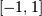numpy.polynomial.legendre.legweight¶

numpy.polynomial.legendre.legweight(x)[source]

Weight function of the Legendre polynomials.

The weight function isand the interval of integration is. The Legendre polynomials are orthogonal, but not normalized, with respect to this weight function.

Parameters : x : array_like Values at which the weight function will be computed. w : ndarray The weight function at x.

Notes

Previous topic

numpy.polynomial.legendre.leggauss

Next topic

numpy.polynomial.legendre.legcompanion